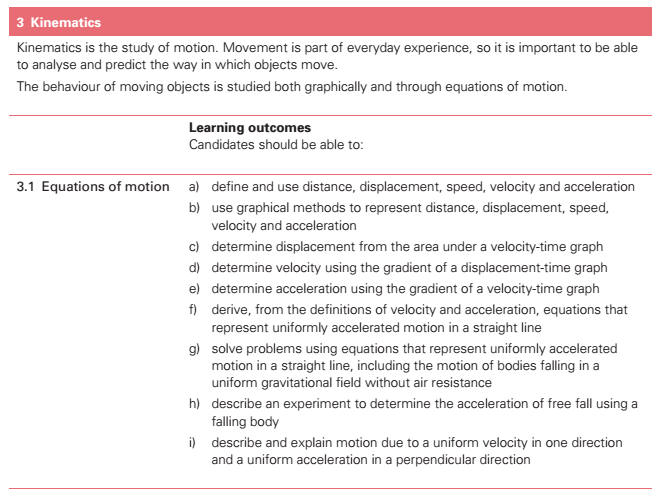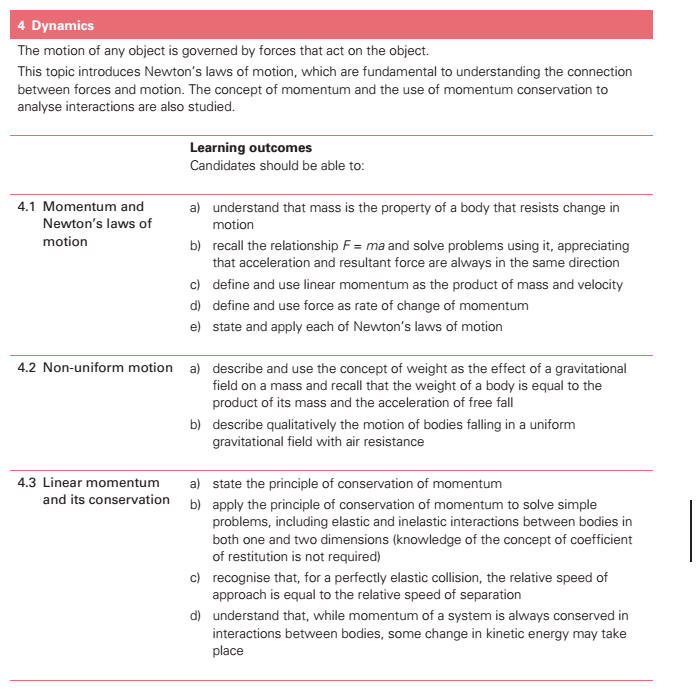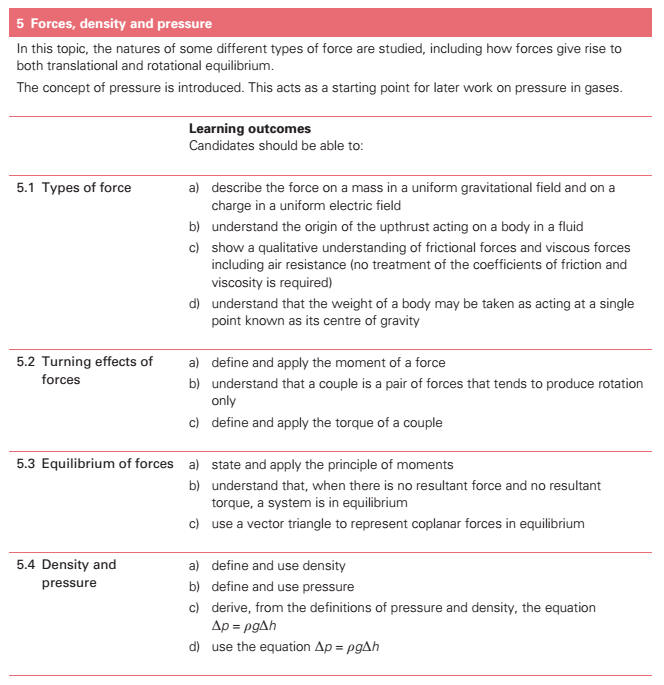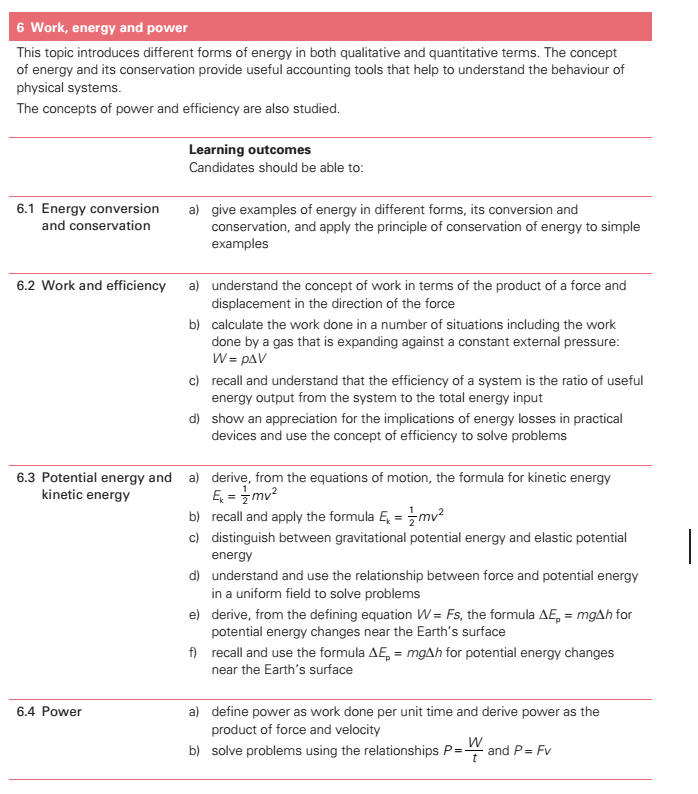3. Kinematics (a) define displacement, speed, velocity and acceleration[]. * (b) use graphical methods to represent displacement, speed, velocity and acceleration[]. * (c) find displacement[][] from the area under a velocity-time graph. * (d) use the slope of a displacement-time graph to find the velocity. * (e) use the slope of a velocity-time graph to find the acceleration. (f) derive, from the definitions of velocity and acceleration, equations which represent uniformly accelerated motion in a straight line. * (g) solve problems using equations which represent uniformly accelerated motion in a straight line, including the motion of bodies falling in a uniform gravitational field without air resistance. (h) recall that the weight of a body is equal to the product of its mass and the acceleration[] of free fall. * (i) describe an experiment to determine the acceleration[] of free fall using a falling body. (j) describe qualitatively the motion of bodies falling in a uniform gravitational field with air (k) describe and explain motion due to a uniform velocity in one direction and a uniform acceleration[] in a perpendicular direction. 4. Dynamics (a) state each of Newtons laws of motion. (b) show an understanding that mass is the property of a body which resists change in motion. (c) describe and use the concept of weight as the effect of a gravitational field on a mass. (d) define linear momentum as the product of mass and velocity. (e) define force as rate of change of momentum. * (f) recall and solve problems using the relationship F = ma, appreciating that acceleration[] and force are always in the same direction. (g) state the principle of conservation of momentum. * (h) apply the principle of conservation of momentum[] to solve simple problems including elastic and inelastic interactions between two bodies in one dimension. (Knowledge of the concept of coefficient of restitution is not required.) * (i) recognise that, for a perfectly elastic collision, the relative speed of approach is equal to the relative speed of separation. * (j) show an understanding that, whilst momentum[] of a system is always conserved in interactions between bodies, some change in kinetic energy[] usually takes place. 5. Forces, density and pressure (a) describe the forces on mass and charge in uniform gravitational and electric fields[], as appropriate. (b) show an understanding of the origin of the upthrust[] acting on a body in a fluid. (c) show a qualitative understanding of frictional forces and viscous forces including air resistance. (No treatment of the coefficients of friction[] and viscosity[] is required.) (d) use a vector triangle to represent forces in equilibrium[]. (e) show an understanding that the weight of a body may be taken as acting at a single point known as its centre of gravity[]. (f) show an understanding that a couple[] is a pair of forces which tends to produce rotation only. (g) define and apply the moment of a force and the torque of a couple. (h) show an understanding that, when there is no resultant force[] and no resultant torque[], a system is in equilibrium[]. (i) apply the principle of moments. (a) define the term density[][]. (f) define the term pressure[] (g) derive, from the definitions of pressure[] and density, the equation p = ρgh. (h) use the equation p = ρgh. 6. Work, Energy, Power[] (a) give examples of energy in different forms, its conversion and conservation, and apply the principle of energy conservation to simple examples. (b) show an understanding of the concept of work in terms of the product of a force and displacement[][] in the direction of the force. * (c) calculate the work done in a number of situations including the work done by a gas which is expanding against a constant external pressure[]: W = p ∆V. (d) derive, from the equations of motion, the formula Ek = ½mv2. (e) recall and apply the formula Ek = ½mv2. (f) distinguish between gravitational potential energy[], electric potential energy and elastic * (g) show an understanding and use the relationship between force and potential energy[] in a uniform field to solve problems. (h) derive, from the defining equation W = Fs, the formula Ep = mgh for potential energy changes near the Earths surface. (i) recall and use the formula Ep = mgh for potential energy[] changes near the Earths surface. (j) show an understanding of the concept of internal energy[]. (k) show an appreciation for the implications of energy losses in practical devices and use the concept of efficiency[] to solve problems. * (l) define power[] as work done per unit time and derive power[] as the product of force and velocity.